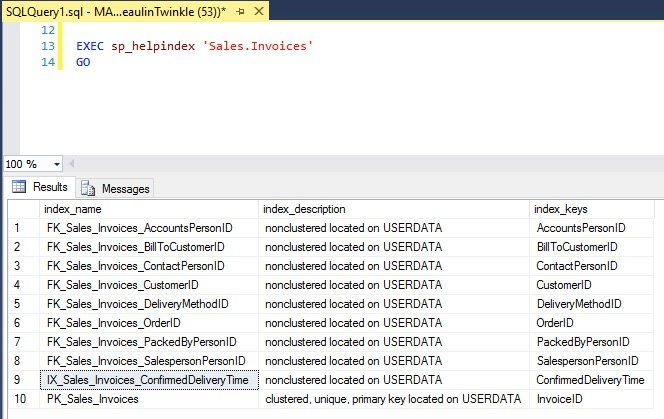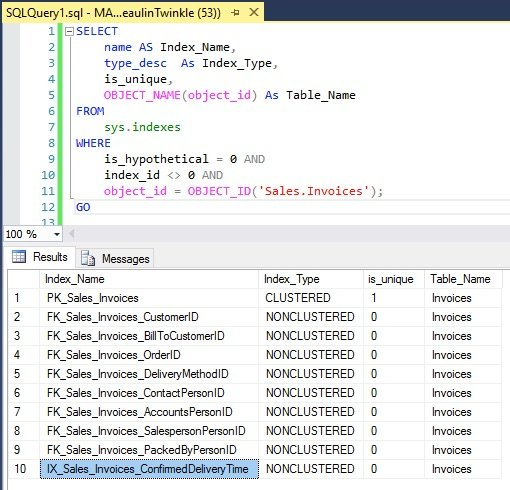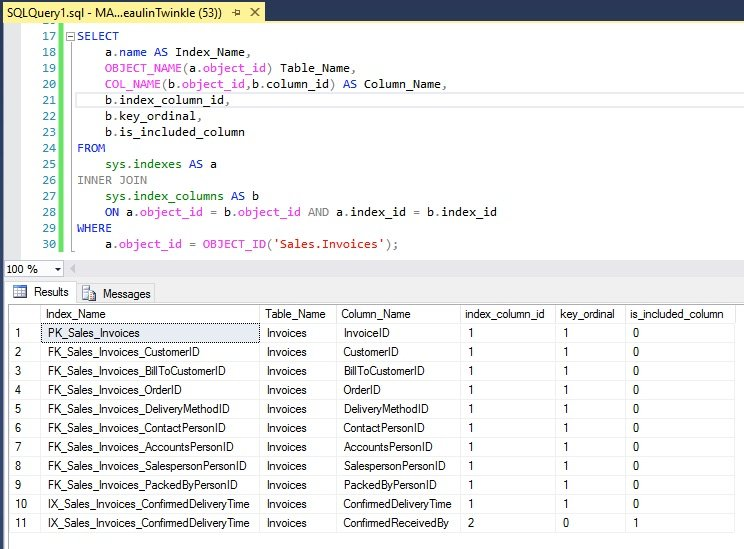# Find Indexes On A Table In SQL Server

There are several methods to find indexes on a table. The methods include using system stored procedure sp_helpindex, system catalog views like sys.indexes or sys.index_columns. We will see these methods one by one.

## 1. Find Indexes on a Table Using SP_HELPINDEX

sp_helpindex is a system stored procedure which lists the information of all the indexes on a table or view. This is the easiest method to find the indexes in a table. sp_helpindex returns the name of the index, description of the index and the name of the column on which the index was created.

### Syntax

```EXEC sp_helpindex '[[[SCHEMA-NAME.TABLE-NAME]]]'
GO
```

### Example## 2. Using SYS.INDEXES

The sys.indexes system catalog view returns all the indexes of the table or view or table valued function. If you want to list down the indexes on a table alone, then you can filter the view using the object_id of the table. Here is the syntax for using the sys.indexes view to list the indexes of a table. In this example, I’ve filtered out the hypothetical index using the WHERE clause condition is_hypothetical = 0. If you think you need to see the hypothetical index, then ignore this condition

### Syntax

```SELECT
name AS Index_Name,
type_desc  As Index_Type,
is_unique,
OBJECT_NAME(object_id) As Table_Name
FROM
sys.indexes
WHERE
is_hypothetical = 0 AND
index_id != 0 AND
object_id = OBJECT_ID('[[[SCHEMA-NAME.TABLE-NAME]]]');
GO
```

### Example## 3. Using SYS.INDEX_COLUMNS

This method is an extension of the earlier sys.indexes method. Here we are joining another system catalog view, sys.index_columns to get the name of the column or columns the index was created or included. This will be helpful to see the column names along with the index name.

### Syntax

```SELECT
a.name AS Index_Name,
OBJECT_NAME(a.object_id),
COL_NAME(b.object_id,b.column_id) AS Column_Name,
b.index_column_id,
b.key_ordinal,
b.is_included_column
FROM
sys.indexes AS a
INNER JOIN
sys.index_columns AS b
ON a.object_id = b.object_id AND a.index_id = b.index_id
WHERE
a.is_hypothetical = 0 AND
a.object_id = OBJECT_ID('[[[SCHEMA-NAME.TABLE-NAME]]]');
```

### Example### Reference

• Querying the SQL Server system catalog FAQ at MSDN.

### Related Articles & Tools

This site uses Akismet to reduce spam. Learn how your comment data is processed.